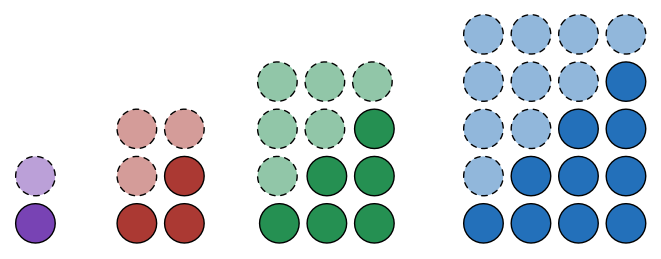# Triangular Numbers 2 – Rectangles?

Algebra Level 1If we double a triangular number, we can turn it into a rectangle.

How tall is the rectangle made from the $6^{th}$ triangular number? (It will have a width of 6.)

×